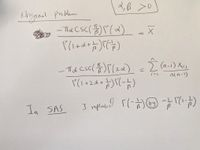## LEVMAR Optimization

Hello

I am trying to estimate my parameters by solving a system of nonlinear equations when the sample size is small. The irony is that the code works if the number of simulations is less than or equal to 10!!!

I tried to use the method from the previous question on this group, that is to solve the log functions instead of the functions themselves but this didn't work for me. Instead, I keep getting errors like "

ERROR: LEVMAR Optimization cannot be completed.
ERROR: The function value of the objective function cannot be computed during the optimization process.
ERROR: Execution error as noted previously. (rc=100)

Also, errors such as the argument of the log are negative. But if the problem is with a negative argument why it is only a problem when n is small?

For clarifications, I am attaching the image of my original problem1 ACCEPTED SOLUTION

Accepted Solutions

## Re: LEVMAR Optimization

Simulation studies with small samples might not converge because the statistics exhibit greater variation when the random samples are small. For example, the standard error of the mean and standard deviation of a small sample is greater than for a larger sample.   This means that the sample-based estimates might be quite far away from the population parameters, which can lead to lack-of-convergence if, for example, you are using the method of moments to estimate parameters.

I think there is a problem with your handwritten notes. Your statement, "in SAS, I replaced...." is not correct. The correct identity is  z*Gamma(z) = Gamma(1+z).

2 REPLIES 2

## Re: LEVMAR Optimization

Simulation studies with small samples might not converge because the statistics exhibit greater variation when the random samples are small. For example, the standard error of the mean and standard deviation of a small sample is greater than for a larger sample.   This means that the sample-based estimates might be quite far away from the population parameters, which can lead to lack-of-convergence if, for example, you are using the method of moments to estimate parameters.

I think there is a problem with your handwritten notes. Your statement, "in SAS, I replaced...." is not correct. The correct identity is  z*Gamma(z) = Gamma(1+z).

From The DO Loop